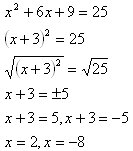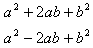Introduction

An equation in which one side is a perfect square trinomial can be easily solved by taking the square root of each side. Consider the example below.When one side of the equation is not already a perfect square trinomial, the process taken to create a perfect square trinomial is called completing the square. Recall that a perfect square trinomial comes in one of two forms:Listen